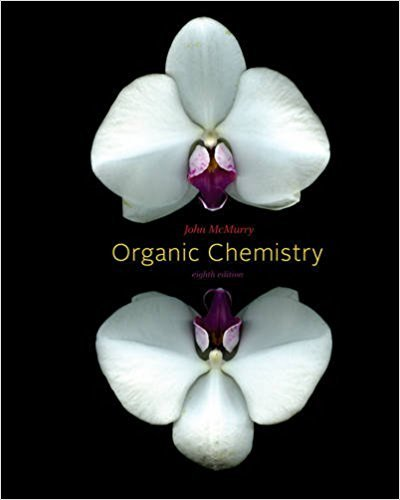×
Get Full Access to Organic Chemistry - 8 Edition - Chapter 9 - Problem 9.23
Get Full Access to Organic Chemistry - 8 Edition - Chapter 9 - Problem 9.23

×

# Predict the products from reaction of 5-decyne with the following reagents: (a) H2ISBN: 9780840054449 463

## Solution for problem 9.23 Chapter 9

Organic Chemistry | 8th Edition

• Textbook Solutions
• 2901 Step-by-step solutions solved by professors and subject experts
• Get 24/7 help from StudySoup virtual teaching assistantsOrganic Chemistry | 8th Edition

4 5 1 247 Reviews
17
5
Problem 9.23

Predict the products from reaction of 5-decyne with the following reagents: (a) H2, Lindlar catalyst (b) Li in NH3 (c) 1 equiv Br2 (d) BH3 in THF, then H2O2, OH2 (e) H2O, H2SO4, HgSO4 (f) Excess H2, Pd/C catalyst

Step-by-Step Solution:
Step 1 of 3

Practice Questions for Stocks and Bonds and Explanation ( Part 2 ) Explaining Important Most important 11) The yield to maturity on a discount bond is : a. equal to the coupon rate. b. greater than the coupon rate. c. less than the coupon rate Answer B : discount bond means they need to pay you more, when we have the yield to maturity > Coupon rate => price bond < par value 12) The dividend growth model can be used to value the stock of firms which pay which type ofdividends I. constant annual dividend II. annual dividend with a constant increasing rate of growth III. zero dividend A. I only B. II only C. I and II only D. I, II, and III only Answer C : Based on the basic formula : Po = Do ( 1 + g) / (R-g) = D1 / (R-g) 13) Which one of the foll

Step 2 of 3

Step 3 of 3

##### ISBN: 9780840054449

Unlock Textbook Solution